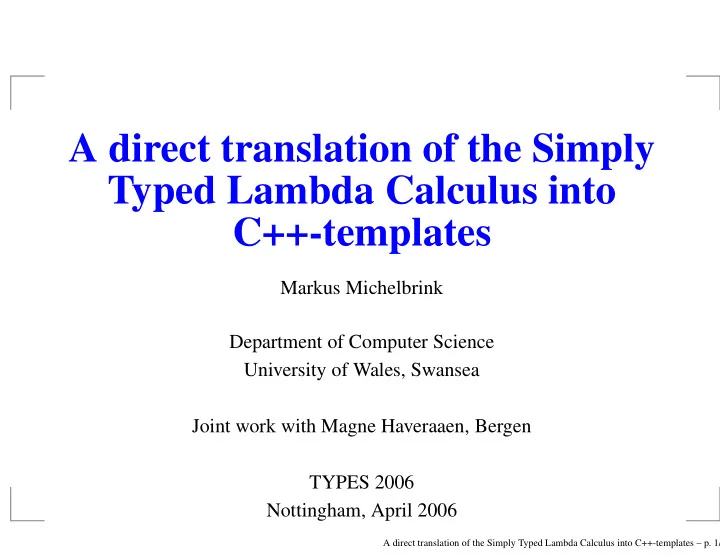# A direct translation of the Simply Typed Lambda Calculus into - PowerPoint PPT Presentation

## A direct translation of the Simply Typed Lambda Calculus into C++-templates Markus Michelbrink Department of Computer Science University of Wales, Swansea Joint work with Magne Haveraaen, Bergen TYPES 2006 Nottingham, April 2006 A direct

1. A direct translation of the Simply Typed Lambda Calculus into C++-templates Markus Michelbrink Department of Computer Science University of Wales, Swansea Joint work with Magne Haveraaen, Bergen TYPES 2006 Nottingham, April 2006 A direct translation of the Simply Typed Lambda Calculus into C++-templates – p. 1/9

2. Basic ideas One ground type: ⋆ ≈ class template < class X_s > struct someClass {...;}; is of type ⋆ → ⋆ , template < template < class X_s > class X_nss > struct someClass {...;}; is of type ( ⋆ → ⋆ ) → ⋆ , template < template < class X_s > class X_nss, class X_s > struct someClass {...;}; is of type ( ⋆ → ⋆ ) → ⋆ → ⋆ , ... A direct translation of the Simply Typed Lambda Calculus into C++-templates – p. 2/9

3. Basic ideas η -expand and abstract from free variables: v s : ⋆ λv s .v s : ⋆ → ⋆ �→ v nss : ⋆ → ⋆ λv nss , v s .v nss v s : ( ⋆ → ⋆ ) → ⋆ → ⋆ �→ The variable v s : ⋆ translates to template < class X_s > struct V_s {typedef typename X_s::result result;}; The variable v nss ∼ βη λv s .v nss v s : ⋆ → ⋆ translates to template < template < class X_s > class X_nss, class X_s > struct V_nss {typedef typename X_nss<X_s>::result result;}; A direct translation of the Simply Typed Lambda Calculus into C++-templates – p. 3/9

4. Basic ideas Application is instantiation: The term v nss v s : ⋆ translates to template < template < class X_s > class X_nss, class X_s > struct V_nssVs {typedef typename V_nss<X_nss,V_s<X_s>>::result result;}; If v ∈ FV ( t ) : abstraction does not change anything. E.g. λv s .v nss v s : ⋆ → ⋆ translates to the template above. If v ∈ FV ( t ) : add a further parameter. E.g. λw s .v nss v s : ⋆ translates to template < class Y_s, template < class X_s > class X_nss, class X_s > struct V_nssVs {typedef typename V_nss<X_nss,V_s<X_s>>::result result;}; A direct translation of the Simply Typed Lambda Calculus into C++-templates – p. 4/9

5. Basic ideas Constants of ground type are given by struct constant_int {typedef int result;}; struct constant_double {typedef double result;}; Theorem: "The translation of every closed term of ground type evaluates to the value of the closed term." A direct translation of the Simply Typed Lambda Calculus into C++-templates – p. 5/9

6. λ into λ T ( v i : A 1 . . . A m → ⋆ ) = λv i +1 , . . . , v i + m .v i v i +1 . . . v i + m T ( t 0 ) = λv 1 . . . v m .t ′ 0 then λv 2 . . . v m .t ′ T ( t 0 t 1 ) = [ v 1 := T ( t 1 )] 0 � �� � some α -conversion T ( λv.t ) = λv. T ( t ) for v ∈ FV ( t ) T ( λv.t ) = λv new . T ( t ) for v �∈ FV ( t ) where A 1 . . . A m → ⋆ := A 1 → ( A 2 . . . ( A m → ⋆ ) . . . ) . A direct translation of the Simply Typed Lambda Calculus into C++-templates – p. 6/9

7. Properties T is welldefined on welltyped terms i.e. t : A → B ⇒ T ( t ) = λv : A.t ′ for some t ′ . T ( t ) is welltyped and Type ( T ( t )) = Type ( t ) FV ( T ( t )) = FV ( t ) T ( t ) ∼ αβη t Adding constants of ground type c 0 , c 1 , . . . : ⋆ we get T ( t ) ∼ β t for every closed term t : ⋆ . A direct translation of the Simply Typed Lambda Calculus into C++-templates – p. 7/9

8. What it is ... In terms of the lambda cube we have ( ✷ , ✷ ) but without ( ⋆, ⋆ ) ! Where ⋆ ≈ class . Do we have more? A direct translation of the Simply Typed Lambda Calculus into C++-templates – p. 8/9

9. What it is ... We can build template < int i > struct someClass {enum ...}; ≈ ( i : int ) → class . Dependent types ( ⋆, ✷ ) ? Function templates are build similar to class templates: template < int i > int justTheIdentity() {return i;}; ≈ int → int . Simple Types ( ⋆, ⋆ ) ? template < class X > X someFunction() {...}; ≈ ( X : class ) → X . Polymorphism ( ✷ , ⋆ ) ? No, we can not use them as parameters! A direct translation of the Simply Typed Lambda Calculus into C++-templates – p. 9/9

More recommend